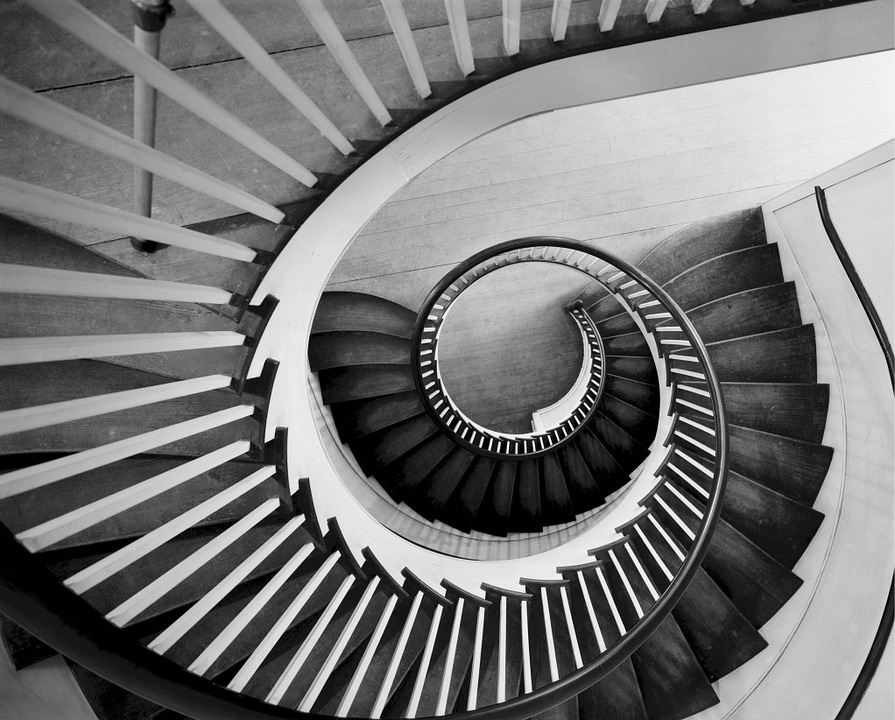## ________________

Numerical work is always on the math team’s agenda.

## ________________### Staircase Numbers

Consider A = 10,203,040,506,070,809 and B = 90,807,060,504,030,201.

Write A as the product of two factors, one having 10 digits and the other having 7 digits.
Write B as the product of two factors, one having 9 digits and the other having 8 digits.

### Arithmetical Question

Is the number of seconds in 6 weeks more, less, or the same as 10 factorial = 1 × 2 × 3 × 4 × 5 × 6 × 7 × 8 × 9 × 10 = 3,628,800?

## Solutions to week 142

In A Golden Set, the probability is 2.980232… × 10-7. In Combining Rule, x = 12.

A Golden Set answer explained:
The probability of either player winning the first 24 points of a set is p = (1/2)23 = 1.192092896… × 10-7. Half of the matches played will take 2 sets and half of them will take 3 sets. The probability of no golden set in a 2-set match is (1-p)2 so that the probability of a golden set in a 2-set match is P2 = 2p-p2. The probability of no golden set for a 3-set match is (1-p)3, so the probability of a golden set in a 3-set match is P3 = 3p – 3p2 + p3. The probability of a golden set in a women’s match is then Pw = (P2 + P3)/2 = 5p/2 – 2p2 + p3/2 ≈ 2.980232 × 10-7.

Combining Rule answer explained:
The combination rule is not subtraction but the sum of the digits of the two numbers, giving x = 2 + 1 + 3 + 6 + = 12.

## Recent Weeks

Links to all of the puzzles and solutions are on the Complete Varsity Math page.

Come back next week for answers and more puzzles.